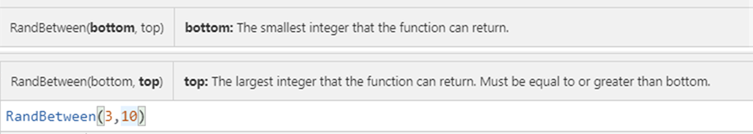# New Power Apps function - RandBetweenThe `Rand()` function returns random number between 0-1.

To get random number with arbitrary min. and max. value, you can use following expression using Rand():

`min + (max-min)*Rand()`

Alternatively, using `Shuffle(...)` and `Sequence(....)`, it returns also random number (integer):

`First(Shuffle(Sequence(max-min,min))).Value`

The New function available in Power Apps today, RandBetween(min, max) provides random integer between min. and max.

This function works same as `rand(...)` function in Power Automate.

`ex: RandBetween(3,10) --> Either value in  [3,4,5,6,7,8,9,10]`# Example1

To pick up record in random sequence, `RandBetween(1, CountRows(..))` works as index of random record.

`Last(FirstN( datasource, RandBetween(1,CountRows(datasource))))`

This is equivalent as

`First(Shuffle(datasource))`

# Example2

Since RandBetween only provides random integer, if maker needs random value with specific precision, e.g. random number between -3.21 and 2.34, it will be expressed as

`RandBetween(min*Power(10,precision),max*Power(10,precision))/Power(10,precision)`

That's all!